# Analytical solution of sea water steady magneto-hydrodynamic equations subjected to stretching sheet under induced magnetic field and heat transfer

Analytical solution of sea water steady magneto-hydrodynamic equations subjected to stretching sheet under induced magnetic field and heat transfer
Faculty of Science, Department of Mathematics, Shebin Elkom 32511, Egypt

Faculty of Science, Department of Physics, Shebin Elkom 32511, Egypt

Corresponding Author Email:
aly.abourabia@science.menofia.edu.eg
Page:
141-151
|
DOI:
https://doi.org/10.18280/mmep.060119
13 November 2018
|
Accepted:
26 February 2019
|
Published:
31 March 2019
| Citation

OPEN ACCESS

Abstract:

The aim of this study is to investigate the problem of steady magneto-hydrodynamic (MHD) flow of an in-compressible viscous fluid over a stretching sheet. This system of nonlinear differential equations is solved analytically using the homogeneous balance method where the induced magnetic field and heat transfer are taken into account. Sea water is the working fluid in our problem. Through this study, it was found that the re­sulting solutions include the non-dimen­sional temperature, velocity, induced magnetic field functions and the ratio between kinetic and magnetic energies depend on different governing parameters. The obtained solutions are plotted at selected values of the prevailing parameters including the mag­netic parameter and the ratio between the straining velocity of the stagnation point flow and the stretching velocity of the sheet. The results indicate that all these quantities are damping far from the stretching source. The findings of this research may serve as a contribution for studying the thermodynamic irreversible processes that is responsible for consuming the energy within the system under scope of the study. This aspect is attained through representing the non-dimensional entropy generation and Bejan numbers at the selected prevailing parameters.

Keywords:

Bejan number, entropy generation, heat transfer, homogeneous balance method, incompressible MHD equations, seawater data, stretched sheets

1. Introduction

The study of stretching layers and thin sheets represents actual subject in characterizing seawater, see Figure A. The motion of seawater, which is a conductor, in the earth’s magnetic field induces a current density. This current density, in turn, induces its own magnetic field . So the study of the magneto-hydrodynamic (MHD) flow of an electrically conducting fluid due to the stretching sheet is important in modern applications and working proc­esses, which is governed by the structure of the boundary layer near the stretching sheets in several media [2-4].

In geophysics, a pioneering series of works constitute a review of theoretical models for electromagnetic induction in the ocean has been conducted by Ashour [5-8] concerning stretching sheets. McKirdy, D.M.in  demonstrated, via comprehensive works of several scien­tists that a model in which an oceanic strip and a perfectly conducting mantle were connected by a crust of small, but non-zero, conductivity and that electric currents could be made to flow along the ocean, down through the crust, back along the mantle and return upwards into the ocean through the crust, for the case of H-polarization. In this situation the in­ducing magnetic field is parallel to the strike, with the result that no induced magnetic field can be detected outside the conductor, provided the conductivity structure is strictly two-dimensional. The horizontal magnetic field below the sea floor was calculated and the effect of return currents in the mantle was shown to be important provided that the width of the ocean was greater than two skin-depths in the crust. Hurley, D. G. and, Siew, P. F. in  considered that the electromagnetic response of a thin conducting sheet in the low frequency limit when the depth of penetration of the primary magnetic field is much greater than the thickness of the sheet is of interest in a number of branches of geophysics including ionospheres’ physics and geophysical. Al-Odat et al.,  presented numerical solutions for the thermal boundary layer of an exponentially continuous stretching surface in the presence of a magnetic field with variable exponential temperature at the surface. Li et al.,  represented multiple solutions of laminar flow in channels with a transverse magnetic field. Kumari et al.  investigated the MHD flow and heat transfer over a stretching surface by considering the effect of the induced magnetic field. The principle of electromagnetic induction due to water flow is long known and was first described by Faraday , Mahapatra and Gupta  reconsidered the stagnation point flow problem towards a stretching sheet taking different stretching and straining velocities and they observed two different kinds of boundary layer near the sheet depending on the ratio of the stretching and straining constant.

To date, little theoretical works had been done on finding an exact solution for the MHD flow and heat transfer over a stretching surface by considering the effect of the induced magnetic field. Also, the irreversible thermodynamic point of view was almost not contributed to many of the previous studies. To solve these defects, this paper establishes a model based on a system of nonlinear differential equations, and applies it to simulate the MHD flow under the previously mentioned conditions, with the aim to find an exact method of solution for those equations. The findings shed new light on studying the induced magnetic field due to the flow of sea water through the Earth’s magnetic field (motional induction) and studying the entropy generation within the system under scope.

While fresh water has a very low conductivity, a salt concentration of about 35 grams per litre turns the oceans into good conductors. When using globally uniform sea water conductivity, the signals of the motional induced magnetic field at sea level due to global ocean circulation ranges from -5 to 4  Nano-Tesla at sea level altitude. Mean values over the simulation period are in the range of 4 to 3 Nano-Tesla .

The remainder of this paper is organized as follows: Section 2 introduces the physical problem and basic equations, Section 3 describes the method of solution and Section 4 represents the discussion and conclusion.

2. The Physical Problem and Basic Equations

In our problem a suitable set of equations for steady 2D flow motion of an in-compressible electrically conducting fluid (sea water) is concerned, subjected to a stretching surface in its own plane with a velocity proportional to the distance from the stagnation-point. We assume that the stretching sheet to be of infinitesimal thickness ($δ$). The medium on both sides is assumed to be non-conducting . The induced magnetic field effect and heat transfer are taken into account. The flow geometry after making a modification according to the physical situation of the problem with the applied geomagnetic field is shown in Figure A. The basic equations for the flow of a viscous and electrically conducting fluid are given by Cowling et al., . Applying the boundary layer approximation , the equations for the problem under consideration are :

$\frac{\partial u}{\partial x}+\frac{\partial v}{\partial y}=0$ (1)

$\frac{\partial {{H}_{1}}}{\partial x}+\frac{\partial {{H}_{2}}}{\partial y}=0$ (2)

\begin{align} & u\frac{\partial u}{\partial x}+v\frac{\partial u}{\partial y}-\frac{{{\mu }_{0}}}{4\pi \rho }({{H}_{1}}\frac{\partial {{H}_{1}}}{\partial x}+{{H}_{2}}\frac{\partial {{H}_{1}}}{\partial y})= \\& ({{u}_{e}}\frac{d{{u}_{e}}}{dx}-\frac{{{\mu }_{0}}{{H}_{e}}}{4\pi \rho }\frac{d{{H}_{e}}}{dx})+\nu \frac{{{\partial }^{2}}u}{\partial {{y}^{2}}} \\\end{align} (3)

$u\frac{\partial {{H}_{1}}}{\partial x}+v\frac{\partial {{H}_{1}}}{\partial y}-{{H}_{1}}\frac{\partial u}{\partial x}-{{H}_{2}}\frac{\partial u}{\partial y}={{\mu }_{e}}\frac{{{\partial }^{2}}{{H}_{1}}}{\partial {{y}^{2}}}$ (4)

$u\frac{\partial T}{\partial x}+v\frac{\partial T}{\partial y}=\alpha \frac{{{\partial }^{2}}T}{\partial {{y}^{2}}}$ (5)

The boundary conditions of Eq. (1-5) are as follows:

$v=0,u={{u}_{w}}(x)=cx,\frac{\partial {{H}_{1}}}{\partial y}=0,{{H}_{2}}=0,T={{T}_{w}}\text{ }at\text{ }y=0$

$u={{u}_{e}}(x)=ax,{{H}_{1}}={{H}_{e}}(x)={{H}_{0}}x,T={{T}_{\infty }}\text{ }as\text{ }y\to \infty$

## 1.png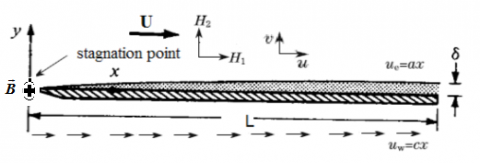Figure 1. The flow geometry of the problem 

Applying the following similarity transformations [20, 21] to the basic equations 

$\eta ={{(\frac{c}{\nu })}^{\frac{1}{2}}}y,\psi (x,y)={{(c\nu )}^{\frac{1}{2}}}xf(\eta )$ (6)

$\phi (x,y)={{H}_{0}}{{(\frac{c}{\nu })}^{\frac{1}{2}}}xg(\eta )$ (7)

$\theta =\frac{T-{{T}_{\infty }}}{{{T}_{w}}-{{T}_{\infty }}}=\frac{T-{{T}_{\infty }}}{\Delta T}$ (8)

ue(x)=ax, uw (x)=cx (9)

${{H}_{0}}={{H}_{e}}x$ (10)

Thus;

$u=\frac{\partial \psi }{\partial y}=\frac{\partial \psi }{\partial \eta }\frac{\partial \eta }{\partial y}=cx\frac{d\text{ }f}{d\eta }$ (11)

$v=-\frac{\partial \psi }{\partial x}=-{{(c\nu )}^{\frac{1}{2}}}f(\eta )$ (12)

${{H}_{1}}=\frac{\partial \phi }{\partial y}=\frac{\partial \phi }{\partial \eta }\frac{\partial \eta }{\partial y}={{(\frac{\nu }{c})}^{\frac{1}{2}}}{{H}_{0}}x{{(\frac{c}{\nu })}^{\frac{1}{2}}}\frac{dg}{d\eta }$ (13)

${{H}_{2}}=-\frac{\partial \phi }{\partial x}=-{{(\frac{\nu }{c})}^{\frac{1}{2}}}{{H}_{0}}g(\eta )$ (14)

$\frac{\partial u}{\partial y}=\frac{\partial }{\partial y}(\frac{\partial \psi }{\partial y})=cx{{(\frac{c}{\nu })}^{\frac{1}{2}}}\frac{{{d}^{2}}f}{d{{\eta }^{2}}}$ (15)

$\frac{du}{dx}=c\frac{df}{d\eta }$ (16)

$\frac{\partial {{H}_{1}}}{\partial x}={{H}_{0}}\frac{dg}{d\eta }$ (17)

$\frac{\partial {{H}_{1}}}{\partial y}=\frac{\partial }{\partial y}(\frac{\partial \phi }{\partial y})={{H}_{0}}x{{(\frac{c}{\nu })}^{\frac{1}{2}}}\frac{{{d}^{2}}g}{d{{\eta }^{2}}}$ (18)

$\frac{{{\partial }^{2}}{{H}_{1}}}{\partial {{y}^{2}}}=\frac{\partial }{\partial y}(\frac{\partial {{H}_{1}}}{\partial y})={{H}_{0}}x(\frac{c}{\nu })\frac{{{d}^{3}}g}{d{{\eta }^{3}}}$ (19)

$\frac{{{\partial }^{2}}u}{\partial {{y}^{2}}}=\frac{\partial }{\partial y}(\frac{\partial u}{\partial y})=(\frac{{{c}^{2}}}{\nu })x\frac{{{d}^{3}}f}{d{{\eta }^{3}}}$ (20)

$T=({{T}_{w}}-{{T}_{\infty }})\theta (\eta )+{{T}_{\infty }}$ (21)

$\frac{\partial T}{\partial x}=0$ (22)

$\frac{\partial T}{\partial y}=({{T}_{w}}-{{T}_{\infty }}){{(\frac{c}{\nu })}^{\frac{1}{2}}}\frac{d\theta }{d\eta }=\Delta T{{(\frac{c}{\nu })}^{\frac{1}{2}}}\frac{d\theta }{d\eta }$ (23)

$\frac{{{\partial }^{2}}T}{\partial {{y}^{2}}}=\frac{\partial }{\partial y}(\frac{\partial T}{\partial y})=\Delta T\frac{c}{\nu }\frac{{{d}^{2}}\theta }{d{{\eta }^{2}}}$ (24)

$\frac{d{{u}_{e}}}{dx}=a$ (25)

$\frac{d{{H}_{e}}}{dx}={{H}_{0}}$ (26)

Substituting from Eq. (11-26) into Eq. (3-5), the following system of nonlinear ordinary differential non-dimensional equations is obtained:

${f}'''+f{f}''-{{({f}')}^{2}}+{{(\frac{a}{c})}^{2}}+\beta ({{{g}'}^{2}}-g{g}''-1)=0$ (27)

$\lambda {g}'''+f{g}''-{f}''g=0$ (28)

${\theta }''+\Pr f{\theta }'=0\text{ }$ (29)

The primes denote differentiation with respect to the similarity variable

. Table 1 contains the definitions of the non-dimensional transformation governing parameters .

Table 1. Definitions of the non-dimensional transformation governing parameters

 The parameter Its definition The velocity ratio a/c $\frac{a}{c}=\frac{u_e(x)}{u_w(x)}$ The magnetic parameter $\beta$ $\beta \text{=}\frac{\mu }{4\pi p}{{\left( \frac{{{H}_{0}}}{c} \right)}^{2}}$ The reciprocal magnetic Prandtl number $\lambda$ $\lambda \text{=}\frac{{{\mu }_{e}}}{v}$ Prandtle number Pr ${{\operatorname{P}}_{r}}=\frac{v}{\frac{{{\lambda }_{0}}}{\rho cp}}$

1. The stretching constants c is fixed at a constant value equal to one;

2. As the uniform induced magnetic field H0  is changed, $\beta$  value changes.

The velocity ratio and the magnetic parameter will play a principle role in the study.

3. Method of Solution

In the known literature throughout the majority of the solutions concerning such problems, among them is the present one; the numerical methods are widely applied with various accuracies. Our concern in this study is devoted to introduce an analytical model, which can be considered as advantageous, and reliable, accordingly a comparison is made with the available previous results.

We shall solve Eq. (27-29) by applying the homogeneous balance method  as follows:

A. At first we should verify the homogeneous balance (between the highest order derivative terms with nonlinear terms) to this system:

In Eq. (27): The highest order derivative term is ${f}'''$ gives n+3, while the highest non-linear term is $f{f}''$ gives 2n+2; equating we get n = 1.

In Eq. (28): The highest order derivative term is ${g}'''$ gives n+3, while the highest non-linear term is $f{g}''$ gives 2n +2; equating we get  n = 1.

In Eq. (29): The highest order derivative term is ${\theta }''$  gives n+2, while the highest non-linear term is $f{\theta }'$  gives 2n +1; equating we get  n = 1.

B. Among the possible solutions representing the non-dimensional velocity, induced magnetic field and temperature are the power series truncated to the term corresponding to n=1 as follows :

$f(\eta )=\sum\limits_{n=0}^{1}{{{a}_{n}}}{{Y}^{n}}={{a}_{0}}+{{a}_{1}}Y$ (30)

$g(\eta )=\sum\limits_{n=0}^{1}{{{b}_{n}}}{{Y}^{n}}={{b}_{0}}+{{b}_{1}}Y$ (31)

$\theta (\eta )=\sum\limits_{n=0}^{1}{{{d}_{n}}}{{Y}^{n}}={{d}_{0}}+{{d}_{1}}Y$ (32)

Here,

$Y=tanh(\eta +\in )$ (33)

The constant shift $\in$ shall be appointed.

The boundary conditions are:

f(0)=0, f'(0)=1, g(0)=0, g'(0)=0, $\theta (0)=1$ at $\eta =0$ (34)

${f}'(\infty )=\frac{a}{c}$,${g}'(\infty )=1$, $\theta (\infty )=0$:at $η→∞$ (35)

C. Substituting from Eq. (30-32) into the equation combined by summing up Eq. (27-29) to reduce the number of differential equations, in order to evaluate the unknown coefficients a0, a1, b0, b1, d0, d1 and ϵ;

$\frac{{{a}^{2}}}{{{c}^{2}}}-f'(\eta )+\Pr f(\eta )\theta '(\eta )\text{+}f(\eta )f''(\eta )-g(\eta )f''(\eta )+f(\eta )g''(\eta )+\beta (-1+g'(\eta ))-g(\eta )g''(\eta ))+\theta ''(\eta )+f'''(\eta )+\lambda g'''(\eta )=0$

By substituting Eq. (30-32) into the above equation a number of independent algebraic equations each of which corresponds to a specific power of Y is obtained, they are solved simultaneously (by using symbolic software), so that the unknown coefficients a0, a1, b0, b1 can be evaluated:

${{a}_{0}}=0,{{a}_{1}}=\frac{4{{c}^{4}}{{\lambda }^{2}}+{{A}_{1}}}{4{{c}^{4}}{{\lambda }^{2}}}$ (36)

${{b}_{0}}=\frac{{{d}_{1}}({{B}_{1}}-{{B}_{2}})}{-2\beta {{A}_{3}}{{A}_{2}}}$ (37)

${{b}_{1}}=\frac{\frac{-{{a}^{2}}}{{{c}^{2}}}+\beta }{4\lambda }$ (38)

where,

${{A}_{1}}=\sqrt{{{a}^{4}}{{c}^{4}}\beta {{\lambda }^{2}}-2{{a}^{2}}{{c}^{6}}{{\beta }^{2}}{{\lambda }^{2}}+{{c}^{8}}{{\beta }^{3}}{{\lambda }^{2}}+16{{c}^{8}}{{\lambda }^{4}}}$

${{A}_{2}}=\beta (-{{a}^{2}}(-1+\beta )+{{c}^{2}}(-\beta +{{\beta }^{2}}+8\lambda ))$

${{A}_{3}}=({{a}^{2}}-{{c}^{2}}\beta )$,${{A}_{4}}=(-1+Tanh[\in ])$

${{A}_{5}}=Sech[\in ](-Cosh[\in ]+Sinh[\in ])$

${{B}_{1}}={{a}^{4}}\Pr \beta -2{{a}^{2}}{{c}^{2}}\Pr {{\beta }^{2}}+{{c}^{4}}\Pr {{\beta }^{3}}-8{{a}^{2}}{{c}^{2}}\beta \lambda +4{{a}^{2}}{{c}^{2}}\Pr \beta \lambda +8{{c}^{4}}{{\beta }^{2}}\lambda$

${{B}_{2}}=4{{c}^{4}}\Pr {{\beta }^{2}}\lambda +32{{c}^{4}}{{\lambda }^{2}}-{{A}_{1}}(8+{{{a}^{2}}\Pr \beta }/{{{c}^{2}}\lambda }\;-{\Pr {{\beta }^{2}}}/{\lambda }\;)$

D. Using the boundary conditions ( $\theta (0)=1,\theta (\infty )=0$), the unknown coefficients d0 and d1are therefore evaluated:

${{d}_{0}}=\frac{1}{1-tanh[\in ]}$ (39)

${{d}_{1}}=\frac{-1}{1-tanh[\in ]}$ (40)

E. Using the boundary condition (g=0 at η=0) after substituting the coefficients b0 and b1 into the expression of  g[η], we obtain the unknown $∈$ :

$\in =Arctan(\frac{{{c}^{2}}\lambda ({{B}_{3}}+{{B}_{4}}+{{B}_{5}})}{{{A}_{2}}{{A}_{3}}^{2}})\text{ }$ (41)

where,

${{B}_{3}}={{a}^{4}}\Pr \beta -2{{a}^{2}}{{c}^{2}}\beta (\Pr (\beta -2\lambda )+4\lambda$

${{B}_{4}}={{c}^{4}}(\Pr {{\beta }^{2}}(\beta -4\lambda )+8\lambda ({{\beta }^{2}}+4\lambda ))$

${{B}_{5}}={{A}_{1}}({{a}^{2}}\Pr \beta -{{c}^{2}}\Pr {{\beta }^{2}}-8{{c}^{2}}\lambda )$

F. Substituting Eq. (36-40) into Eq. (30-32) to get the sought variables:

$\theta (\eta )=\frac{1}{1-tanh[\in ]}-\frac{tanh[\in +\eta ]}{1-tanh[\in ]}$ (42)

$f(\eta )=\frac{(4{{c}^{4}}{{\lambda }^{2}}+{{A}_{1}})tanh[\in +\eta ]}{4{{c}^{4}}{{\lambda }^{2}}}$ (43)

$g(\eta )=\frac{{{B}_{6}}+{{B}_{7}}+{{B}_{8}}}{-4{{c}^{2}}\lambda {{A}_{2}}{{A}_{3}}{{A}_{4}}}$ (44)

where,

${{B}_{6}}=-2(-{{a}^{4}}{{c}^{2}}\Pr \beta \lambda +{{a}^{2}}\beta (2{{c}^{4}}\lambda (\Pr (\beta -2\lambda )+4\lambda )-\Pr {{A}_{1}})$

${{B}_{7}}={{c}^{2}}(-{{c}^{4}}\lambda (\Pr {{\beta }^{2}}(\beta -4\lambda )+8\lambda ({{\beta }^{2}}+4\lambda ))$

${{B}_{8}}=(\Pr {{\beta }^{2}}(\beta -4\lambda )+{{A}_{2}}{{A}_{3}}^{2}{{A}_{5}}tanh[\in +\eta ]$

G. Differentiating Eq. (43) and (44) with respect to η to get the normal velocity ${f}'(\eta)$ and the normal induced magnetic field

${g}'(\eta )$ components;

${f}'(\eta )=\frac{(4{{c}^{4}}{{\lambda }^{2}}+{{A}_{1}})Sech{{[\in +\eta ]}^{2}}}{4{{c}^{4}}{{\lambda }^{2}}}$ (45)

${g}'(\eta )=-\frac{{{A}_{3}}{{A}_{5}}Sech{{[\in +\eta ]}^{2}}}{4{{c}^{2}}\lambda {{A}_{4}}}$ (46)

Kinetic energy per unit mass  K.E. and Magnetic energy M.E. and their ratio $Q(\eta )$ can be respectively written as:

$K.E.=\frac{1}{2}({{f}^{2}}+{{{f}'}^{2}})$ (47)

$M.E.=\frac{{{g}^{2}}+{{({g}')}^{2}}}{8\pi }$ (48)

$Q(\eta )=\frac{K.E.}{M.E.}=\frac{4\pi ({{f}^{2}}+{{{{f}'}}^{2}})}{{{g}^{2}}+{{({g}')}^{2}}}$ (49)

H. The Entropy generation

Entropy generation analysis, and specifically, second law efficiency, is an important tool for illustrating the influence of irreversibility within a system on the required energy input, where salinity of water is introduced . In our work, this thermodynamic tool is used to reveal which of the three properties in the system is prevailing for the problem in hand. Indeed, the volumetric entropy generation (which is derived from energy and entropy balances) , for the case of heat and mass transfer in presence of a magnetic field (B) is given in a general form by:

${{\overset{.}{\mathop{S}}\,}_{gen.}}=\frac{{{\lambda }_{0}}{{(\nabla T)}^{2}}}{{{({{{{T}'}}_{0}})}^{2}}}-\frac{1}{{{{{T}'}}_{0}}}\sum{{{\text{J}}_{\gamma \text{i}}}}\nabla {{\kappa }_{i}}+\frac{\mu \overline{\overline{grad{{V}_{d}}}}}{{{T}_{0}}^{\prime }}+{{\frac{{{\sigma }_{e}}\left| \overrightarrow{V}\times \overrightarrow{B} \right|}{{{{{T}'}}_{0}}}}^{2}}$ (50)

where ${{\text{J}}_{\gamma \text{i}}}$, kand Vare mass flux vector of species in phase $\gamma$, the chemical potential of species i and dimensional velocity vector, respectively.

The conductivity of seawater is a globally uniform value of approximately 4 S/m, which is assumed constant in time. It is derived from salinity and temperature, where the temperature is the predominant component . The motion of this conductor in the geomagnetic field creates an electromotive force. This in turn leads to a current density given by ;

$\overrightarrow{J}={{\sigma }_{e}}(\overrightarrow{V}*\overrightarrow{B})={{\sigma }_{e}}{{\mu }_{0}}({{\overrightarrow{V}}_{0}}*{{\overrightarrow{H}}_{0}})={{\sigma }_{e}}{{\mu }_{0}}(u{{H}_{2}}-v{{H}_{1}})\overrightarrow{k}$ (51)

Since velocity is confined to the x-y plane, the current density $J ⃗$ is in the z-direction. This current density creates a secondary induced magnetic field. We are concerned with the heat transfer in the presence of induced magnetic field and according to the problem situation Eq. (50) can be rewritten as

${{\dot{S}}_{gen.}}=\frac{k}{{{(T_{0}^{'})}^{2}}}{{(\frac{\partial T}{\partial x}+\frac{\partial T}{\partial y})}^{2}}+\frac{\mu }{T_{0}^{'}}(2{{\left( \frac{\partial u}{\partial x} \right)}^{2}}+2{{\left( \frac{\partial v}{\partial x} \right)}^{2}}+2{{\left( \frac{\partial u}{\partial x}+\frac{\partial v}{\partial x} \right)}^{2}})+\frac{{{\sigma }_{e}}{{\mu }_{0}}^{2}}{T_{0}^{'}}{{(u{{H}_{2}}-v{{H}_{1}})}^{2}}$ (52)

By applying the similarity transformations (i.e. Eq. (11-24)) to the above equation, we obtain the final expression:

${{\overset{.}{\mathop{S}}\,}_{gen.}}=\frac{{{\lambda }_{0}}c}{{{T}_{0}}^{2}\nu }{{{\theta }'}^{2}}+\frac{4{{c}^{2}}\mu }{{{T}_{0}}\Delta T}{{{f}'}^{2}}+\frac{{{\sigma }_{e}}{{\nu }^{2}}{{\mu }_{0}}^{2}H_{0}^{2}}{{{T}_{0}}\Delta T}{{(f{g}'-{f}'g)}^{2}}$ (53)

Multiplying both sides of Eq. (53) by $\frac{L_4}{(K_B U}$ seeking the non-dimensional form:

${{\bar{\dot{S}}}_{gen}}={{\bar{\dot{S}}}_{h}}+{{\bar{\dot{S}}}_{V}}+{{\bar{\dot{S}}}_{M}}$ (54)

where:

${{\bar{\dot{S}}}_{h}}={{R}_{1}}{{{\theta }'}^{2}};$Corresponds to heat transfer,

${{\bar{\dot{S}}}_{V}}={{R}_{2}}{{{f}'}^{2}};$Corresponds to viscous dissipation,

${{\bar{\dot{S}}}_{M}}={{R}_{3}}{{(f{g}'-{f}'g)}^{2}};$Corresponds to the induced magnetism, and

${{R}_{1}}=\frac{{{L}^{4}}}{{{K}_{B}}U}\frac{{{\lambda }_{0}}c}{{{T}_{0}}^{2}\nu }\text{, }{{R}_{2}}=\frac{{{L}^{4}}}{{{K}_{B}}U}\frac{4{{c}^{2}}\mu }{{{T}_{0}}\Delta T}\text{ , }{{R}_{3}}=\frac{{{L}^{4}}}{{{K}_{B}}U}\frac{{{\sigma }_{e}}{{\mu }_{0}}^{2}{{\nu }^{2}}H_{0}^{2}}{{{T}_{0}}\Delta T}.$

Bejan number:

In Eq. (54) an alternative parameter for irreversibility distributions is the Bejan number (Be) defined in :

$B{{e}_{h}}=\frac{{{{\bar{\dot{S}}}}_{h}}}{{{{\bar{\dot{S}}}}_{h}}+{{{\bar{\dot{S}}}}_{V}}+{{{\bar{\dot{S}}}}_{M}}}$ (55)

$B{{e}_{V}}=\frac{{{{\bar{\dot{S}}}}_{V}}}{{{{\bar{\dot{S}}}}_{h}}+{{{\bar{\dot{S}}}}_{V}}+{{{\bar{\dot{S}}}}_{M}}}$ (56)

$B{{e}_{M}}=\frac{{{{\bar{\dot{S}}}}_{M}}}{{{{\bar{\dot{S}}}}_{h}}+{{{\bar{\dot{S}}}}_{V}}+{{{\bar{\dot{S}}}}_{M}}}$ (57)

4. Discussion and Conclusion

First of all we resort to the estimated temperature profiles to start within our discussion, since the laws of conservation are the main guaranty in any theoretical study to be consistent with experiment. Throughout the data in several references, we adopt a range of the magnetic parameter and the a/c ratio at certain values of the reciprocal of the magnetic Prandtl number (i.e. $\lambda$  = 15828.8) and Prandtl number (i.e. Pr = 7.2) , non-dimensional temperature, velocity and induced magnetic field profiles are shown in Figures (1-10) as functions of $η$ .

The used parameters are given in Table (2) citing the properties of seawater at salinity of 35 g/kg and temperature 200 C (at 1 atmospheric pressure) .

Table 2. The properties of seawater at salinity of 35 g/kg and temperature 200 C (at 1 atmospheric pressure)

 Dynamic viscosity (μ) 1.08*10-3  Pa s Kinematic viscosity ( v ) 1.05*10-6  m2 s-1 Thermal conductivity ( $λ_0$_ 0.596 W/m K Thermal diffusivity ( $\alpha$ ) 1.46*10-7  m2 s-1 Prandtl number (Pr) 7.2 Specific heat capacity (Cp) 3993 J kg-1 K-1 Electrical conductivity ( ${{\sigma }_{e}}$) 4.788 S m-1 Density ( $\rho$ ) 1024.763 kg m-3 Magnetic permeability (μ0) $4\pi \times {{10}^{-7}}$  N A-2

The odd-numbered figures correspond to different values of β at a fixed value of the ratio a/c>>1. Increasing β which corresponds to increasing the induced magnetic field throughout the thermal boundary layer, tends to decrease the non-dimensional temperature  as illustrated in Figure1 at a fixed value of ∆T = Tw - T = 20 K.
An important result is found includes a reduction of heat transfer in electrically conducting fluids, by which  will tend to equilibrium with nonlinear damping to zero as the fluid flows far from the initial point of stretching, in agreement with previous works [20-21, 27]. Figure 3, describes that the longitudinal flow velocity component $f(\eta )$  is damping to vanish monotonically nonlinearly with increasing β as η increases.

## 2.pngFigure 2. Temperature profile for different values of β; a/c=110

Figure 5 gives the behaviour of the transverse flow velocity component f'(η) ; it is in the negative unit scale and damping to zero as it moves far from the stretching surface for all values of β in a descending order. The stagnation points are found along η direction as the flow is far from the stretching sheet surface and the boundary layer edge.

The nonlinear damping of the positive transverse component of the induced magnetic g'(η)  field for all values of β in ascending order is shown in Figure 6.

The same tendency is shown in Figure 5 for the longitudinal component of the induced magnetic field g(η)  for all values of β but in an ascending order.

## 3.pngFigure 3. Velocity profile for different values of β at a/c =110

## 4.pngFigure 4. Velocity profile for different values of β; a/c =110

## 5.pngFigure 5. Profile for different values of β; a/c = 110

In Figure 11 it is observed that the increase in β has a direct increasing effect on the ratio Q(η) , which is always greater than unity, but in a descending damping order starting from η = 0.

The even-numbered figures correspond to different values of the velocity ratio parameter (a/c >>1) at a fixed value of magnetic parameter β. The velocity of the boundary layer thickness decreases with increasing values a/c and also the thermal boundary layer thickness decreases with increasing a/c.

## 6.pngFigure 6. g'(η) Profile for different values of β at a/c = 110

## 7.pngFigure 7. Q(η) at different values of β when a/c = 110

The reduction of the thermal boundary layer and the velocity boundary layer thicknesses is due to the increase of the straining velocity compared to the stretching velocity (i.e. increasing a/c ratio).

## 8.png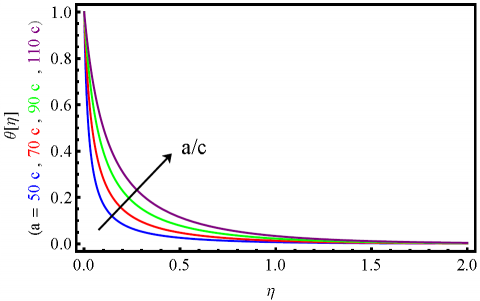Figure 8. Temperature profile for different values of a/c at β = 7.519*10-16

In Figure 8 increasing a/c ratio tends to an increase of the non-dimensional temperature θ(η) for a fixed ∆T. The increase of θ(η), is due to the increase of the fluid bulk temperature T which occurs concurrently with the reduction of the thermal boundary layer thickness.

Also, θ(η) will tend to equilibrium in a nonlinear damping towards zero as the fluid flows far from the initial point of stretching as shown in Figure 8, in agreement with previous works [20-21, 27].

Figure 9, describes that the longitudinal flow velocity component f(η) is damping to vanish monotonically nonlinearly - in ascending values of a/c - as η increases.

Figure 10 gives the behaviour of the transverse flow velocity component f’(η); it is in the negative unit scale and damping to zero as it moves far from the stretching surface for all values of a/c in an ascending order. The stagnation points are found along η direction as the flow is far from the stretching surface and the boundary layer edge.

## 9.pngFigure 9. Velocity profile for different values of a/c at β = 7.519*10-16

The velocity components f(η) and f’(η) decrease is due to the increase of viscous force effect which occurs simultaneously with the thinning of the velocity boundary layer.

## 10.pngFigure 10. Velocity profile for different values of a/c at  β = 7.519*10-16

## 11.pngFigure 11. g(η) profile for different values of a/cat β=7.519 10-16

## 12.png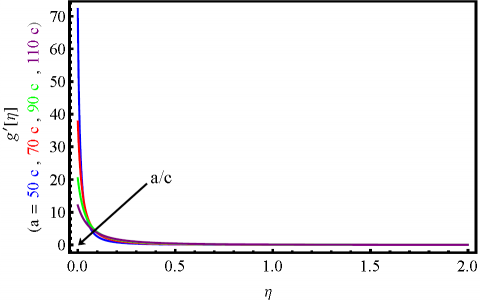Figure 12. g'(η)  Profile for different values of a/c when β = 7.519*10-16

The same tendency is shown in Figure 11 for the longitudinal component of the induced magnetic field g(η)for all values of a/c but in descending order.

The nonlinear damping of the positive transverse component of the induced magnetic g'(η) field for all values of a/c in descending order is shown in Figure 12.

In Figure 13 it is shown that the increase in a/c has a reverse decreasing effect on the ratio Q(η), although is it always greater than unity, but in descending damping order starting from η = 0.

## 13.png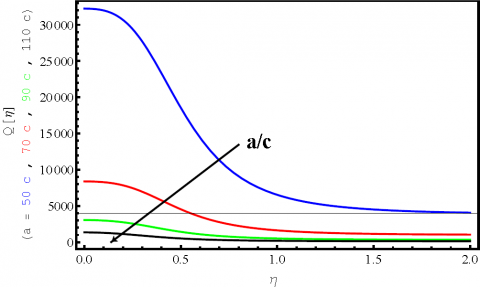Figure 13. Q(η) at different values of a/c at β = 7.519*10-16

The entropy generation due to the heat transfer ($S ̇ ̄_h$) has the major effect on the total entropy generation, it is dominating as seen through the ratios between the three Bejan numbers, which amount to:

Beh: Bev: BeM = 1: 4

10- 6: 9 10- 32

In order to examine the validation of our method of solution together with the physical consistency we are comparing our results with those obtained by Ali, F. M. et al., , where the same problem was solved numerically, considering the air as the working fluid, with the assumption of its incompressibility (Pr= 0.72) at standard conditions. This condition is fulfilled by assuming that air velocity V is about 100 m/s (330 ft/s), making the Mach number Ma< V/c < 0.3, c is the sound speed. So that with the same dimensionless quantities and boundary conditions and by substituting the values of the parameters (a/c, β, Pr) mentioned in [20, 21] in equations (16, 17, 19, 20) of our study. Our results (the figures shown in colour) are compared with those obtained in Ali, F. M. et al.,  (the figures shown in black) as they observed in their original text.

Good agreement is obtained concerning the temperature profile according to the boundary condition i.e. the conservation of thermal en energy for both fluids; see Figures 14, 16 compared to Figures 15, 17 in .

## 14.png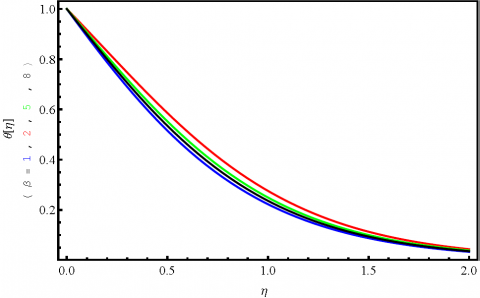Figure 14. Temperature profile for different β at a/c =3

## 15.pngFigure 15. Tempereture profiles for different values of β when a/c=З and Pr=0.72

## 16.pngFigure 16. Temperature profile for different a/c at β = 0.1

By looking to the behaviour of the velocity and magnetic field components; 18 with 19; 20 with 21; 22 with 23; 24 with 27, and 25 with 26, it is found that by our method that they tend to vanish as the fluid flows far from the stretching source, while this is not observed in the corresponding figures cited at  for some values of the ratio a/c and parameter β.

By looking at the behaviour of the velocity and magnetic field components; 16 with 3; 17 with 10; 18 with 5; 19 with 8, it is found that by our method that they tend to vanish as thefluid flows far from the stretching source, while this is not observed in the corresponding figures cited at  for some values of the ratio a/c and parameter β.

## 17.png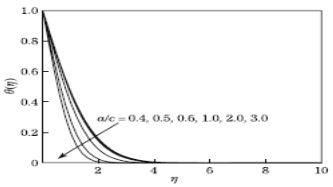Figure 17. Tempereture profiles for different values of a/c when Pr=0.72 and β=0.1

## 18.pngFigure 18. Profile for different values of a/c, β=0.1

## 19.pngFigure 19. g' Profiles for different values of a/c, when Pr=0.72 and β=0.1

## 20.pngFigure 20. g'(η) Profile for different values of β at a/c = 3

## 21.png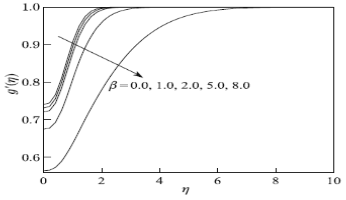Figure 21. g' profiles for different values of β, when a/c=3 and Pr=0.72

## 22.png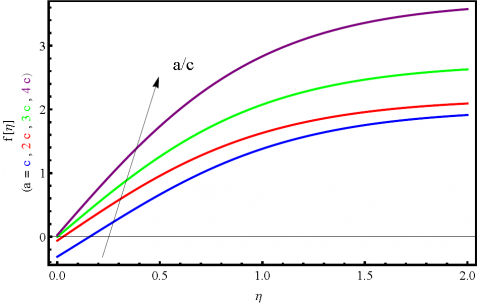Figure 22. Velocity profile for different values of a/c at β =0.1

## 23.pngFigure 23. Variation of f for diferrent values of a/c when Pr=0.72 and β=0.1

## 24.pngFigure 24Velocity profile for different a/c at β = 0.1

## 25.pngFigure 25. Velocity profiles for different values of β when a/c=0.5 and Pr=0.72

## 26.png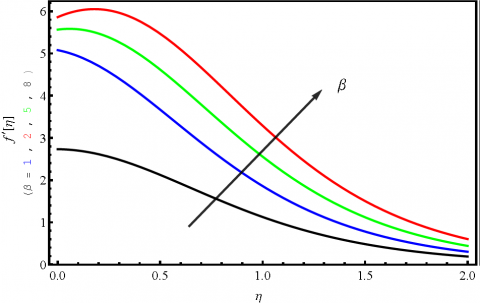Figure 26. Velocity profile for different at a/c = 0.5

## 27.pngFigure 27. Velocity profile for diferrent values of a/c when Pr=0.72 and β=0.1

5. Conclusion

This study considers the flow of an electrically conducting fluid (sea water) past over a stretching sheet where the induced magnetic field is taken into account. We employed the homogenous balance method to solve the flow governing equations by the aid of symbolic software. Some main points are concluded:

• The conservation of energy is maintained in the system, it is demonstrated by the non-dimensional temperature profiles (see Figures1, 2), which agrees with those obtained in the literature.
• It is found that the re­sulting solutions depend on different governing parameters including the mag­netic parameter and the ratio between the straining velocity of the stagnation point flow and the stretching velocity of the sheet.
• The non-dimensional velocity and induced magnetic field are vector components quantities, their behaviours in our study show damping far from the stretching source (see Figures3-12), and they satisfy the physical situation but differ from those obtained by other studies for same values of the controlling parameters.
• Some important physical analysis such as the ratio of the kinetic to magnetic energies within the fluid under study, the development of entropy generation are highlighted by quantifying the corresponding contributions of heat transfer, viscous dissipation and magnetic effects.

We recommend for future studies to extend the findings of this study; first by tackling the nonstationary case to the present problem, second, by applying other approaches for modelling electromagnetic induction in the ocean at a variable seawater conductivity distribution.

Nomenclature
 u, v Velocity components along x and y directions, m.s-1 H1,H2 Induced magnetic field components along x and y directions, A.m-1 H0 Uniform induced magnetic field, A.m-1 He(x) The x-magnetic field at the edge of the boundary layer, A.m-1 T The fluid temperature, K ${{T}_{w}},{{T}_{\infty }}$ The fluid temperature along the wall and at the edge of the boundary layer, K Cp Isobaric specific heat, J. kg-1. K-1 L Characteristic length of the system, m KB Boltzmann constant, J. K-1 U Characteristic velocity of the fluid, m.s-1 Ue(x) The straining velocity of the stagnation point flow, m.s-1 Uw(x) The stretching velocity of the sheet, m.s-1 $f(\eta ),{f}'(\eta )$ Non-dimensional velocities along x and y directions $g(\eta ),{g}'(\eta )$ Non-dimensional induced magnetic fields along x and y directions Pr The Prandtl number R1 , R2 , R3 Irreversibility distribution ratios a, c (>0) The straining and stretching constants

Greek symbols

 $\rho$ Fluid density, kg. m-3 µ dynamic viscosity, kg. m-1.s-1 µ0 The magnetic permeability, N.A-2 µe The magnetic diffusivity, m2 s-1 $\alpha$ The thermal diffusivity, m2 s-1 v The kinematic viscosity, m2 s-1 ${{\sigma }_{e}}$ The electrical conductivity of the fluid, S.m-1 $\theta (\eta )$ Non-dimensional temperature $\eta$ Similarity variable $\psi (x,y)$ Stream function $\phi (x,y)$ Magnetic Stream function $\beta$ The magnetic parameter $\lambda$ The reciprocal magnetic Prandtl number $λ_0$ The thermal conductivity of the fluid, W.m-1. K-1
References

 Davis CA. (1991). Magnetic fields generated by internal ocean seawater motion. M.S.Thesis, Calhoun. Naval Postgraduate School. Monterey, California.

 Kafoussias NG, Tzirtzilakis EE. (2003). Biomagnetic fluid flow over a stretching sheet with non linear temperature dependent magnetization. Zeitschrift für Angewandte Mathematik Und Physik (ZAMP) 54(4): 551–565. https://doi.org/10.1007/s00033-003-1100-5

 Tzirtzilakis EE, Tanoudis GB. (2003). Numerical study of biomagnetic fluid flow over a stretching sheet with heat transfer. International Journal of Numerical Methods for Heat & Fluid Flow 13(7): 830–848. https://doi.org/10.1108/09615530310502055

 Tzirtzilakis EE, Kafoussias NG. (2010). Three-dimensional magnetic fluid boundary layer flow over a linearly stretching sheet. Journal of Heat Transfer 132(1): 011702. https://doi.org/10.1115/1.3194765

 Ashour AA. (1971). Electromagnetic induction in thin finite sheets having conductivity decreasing to zero at the edge, with geomagnetic applications I. Geophysical Journal International 22(4): 417–443. https://doi.org/10.1111/j.1365-246x.1971.tb03610.x

 Asbour AA. (1971). Electromagnetic induction in thin finite sheets having conductivity decreasing to zero at the edge, with geomagnetic applications--II. Geophysical Journal International 25(5) 447–467. https://doi.org/10.1111/j.1365-246x.1971.tb02198.x

 Ashour AA. (1973). Theoretical models for electromagnetic induction in the oceans. Physics of the Earth and Planetary Interiors 7(3): 303–312. https://doi.org/10.1016/0031-9201(73)90056-3

 Ashour AA. (1974). The condition at the surface of a thin conductor in which currents are induced. Geophysical Journal International 36(1): 235–237. https://doi.org/10.1111/j.1365-246x.1974.tb03634.x

 McKirdy DM. (1980). Electromagnetic induction in the earth and oceans. Ph.D. thesis, Faculty of Science of the University of Edinburgh.

 Hurley DG, Siew PF. (1991). A first-order correction to the theory for the electromagnetic response of a thin conducting sheet 1. Geophysical Prospecting 39(4): 527–541. https://doi.org/10.1111/j.1365-2478.1991.tb00326.x

 Al-Odat MQ, Damseh RA, Al-Azab TA. (2006). Thermal boundary layer on an exponentially stretching continuous surface in the presence of magnetic field effect. International Journal of Applied Mechanics and Engineering 11: 289–299.

 Li BT, Zheng LC, Zhang XX. (2009). Multiple solutions of laminar flow in channels with a transverse magnetic field. Chinese Physics Letters 26(9): 094101. https://doi.org/10.1088/0256-307x/26/9/094101

 Kumari M, Takhar HS, Nath G. (1990). MHD flow and heat transfer over a stretching surface with prescribed wall temperature or heat flux. Wärme- Und Stoffübertragung, 25(6): 331–336. https://doi.org/10.1007/bf01811556

 Faraday MV. (1832). Experimental researches in electricity. Philosophical Transactions of the Royal Society of London 122: 125–162. https://doi.org/10.1098/rstl.1832.0006

 Mahapatra TR, Gupta AS. (2001). Magnetohydrodynamic stagnation-point flow towards a stretching sheet. Acta Mechanica 152(1-4): 191–196. https://doi.org/10.1007/bf01176953

 Irrgang C, Saynisch J, Thomas M. (2015). Impact of variable sea-water conductivity on motional induction simulated with an OGCM. Ocean Science Discussions, 12(4): 1869–1891. https://doi.org/10.5194/osd-12-1869-2015

 Cowling TG. (1958). Magnetohydrodynamics. Journal of Fluid Mechanics 3(05): 550-552. https://doi.org/10.1017/s0022112058220181

 Davies TV. (1963). The magneto-hydrodynamic boundary layer in the two-dimensional steady flow past a semi-infinite flat plate. I. Uniform conditions at infinity. Proceedings of the Royal Society of London. Series A. Mathematical and Physical Sciences 273(1355): 496–508. https://doi.org/10.1098/rspa.1963.0105

 Puttkammer PP. (2013). Boundary layer over a flat plate. BSc. Report, University of Twente.

 Ali FM, Nazar R, Arifin NM, Pop I. (2011). MHD stagnation-point flow and heat transfer towards stretching sheet with induced magnetic field. Applied Mathematics and Mechanics 32(4): 409–418. https://doi.org/10.1007/s10483-011-1426-6

 Ferdows M. (2013). Steady laminar boundary layer flow over an impulsively stretching surface enclosed by strong magnetic field. Procedia Engineering 56: 281–286. https://doi.org/10.1016/j.proeng.2013.03.119

 Abourabia AM, El-Danaf TS, Morad AM. (2009). Exact solutions of the hierarchical Korteweg–de Vries equation of microstructured granular materials. Chaos, Solitons & Fractals 41(2): 716–726. https://doi.org/10.1016/j.chaos.2008.03.015

 Mistry KH, McGovern RK, Thiel GP, Summers EK, Zubair SM, Lienhard JH. (2011). Entropy generation analysis of desalination technologies. Entropy 13(10): 1829–1864. https://doi.org/10.3390/e13101829

 Buchdahl HA. (1977). The Thermodynamics of fluid systems. Journal of Fluid Mechanics 83(01): 207. https://doi.org/10.1017/s0022112077211153

 Bejan A. (1982). Second-law analysis in heat transfer and thermal design. Advances in Heat Transfer 1–58. https://doi.org/10.1016/s0065-2717(08)70172-2

 Hill MN. (1963). The Composition of sea-water : comparative and descriptive oceanography / General editor, M.N. Hill. doi:10.5962/bhl.title.16984

 And Cox RA, McCartney MJ, Culkin F. (1970). The specific gravity/salinity/temperature relationship in natural sea water. Deep Sea Research and Oceanographic Abstracts 17(4): 679–689. https://doi.org/10.1016/0011-7471(70)90034-3

 Pazur AKH. (2014). Reduced heat transfer in saltwater by a magnetic field: do oceans have a “geomagnetic brake”? Geophysical & Astrophysical Fluid Dynamics 108(5): 536–552. https://doi.org/10.1080/03091929.2014.934503